/

### The exponential distribution

The exponential distribution describes the time between events if these events follow a Poisson process (i.e. a stochastic process in which events occur continuously and independently of one another). It is also called the negative exponential distribution. It is not the same as the exponential family of distributions.

 Distribution name Exponential distribution Common notation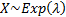Parameters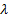= inverse scale (i.e. rate) parameter (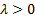) Domain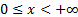Probability density function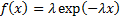Cumulative distribution function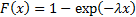Mean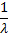Variance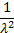Skewness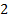(Excess) kurtosis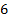Characteristic function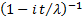Other comments The exponential distribution is a special case of the Gamma distribution, as ifthen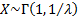.   The mode of an exponential distribution is 0. The quantile function, i.e. the inverse cumulative distribution function, is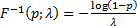.   The non-central moments (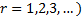are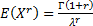. Its median is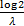.

Nematrian web functions

Functions relating to the above distribution may be accessed via the Nematrian web function library by using a DistributionName of “exponential”. Functions relating to a generalised version of this distribution including an additional location (i.e. shift) parameter may be accessed by using a DistributionName of “exponential2” ”, see also including additional shift and scale parameters. For details of other supported probability distributions see here.